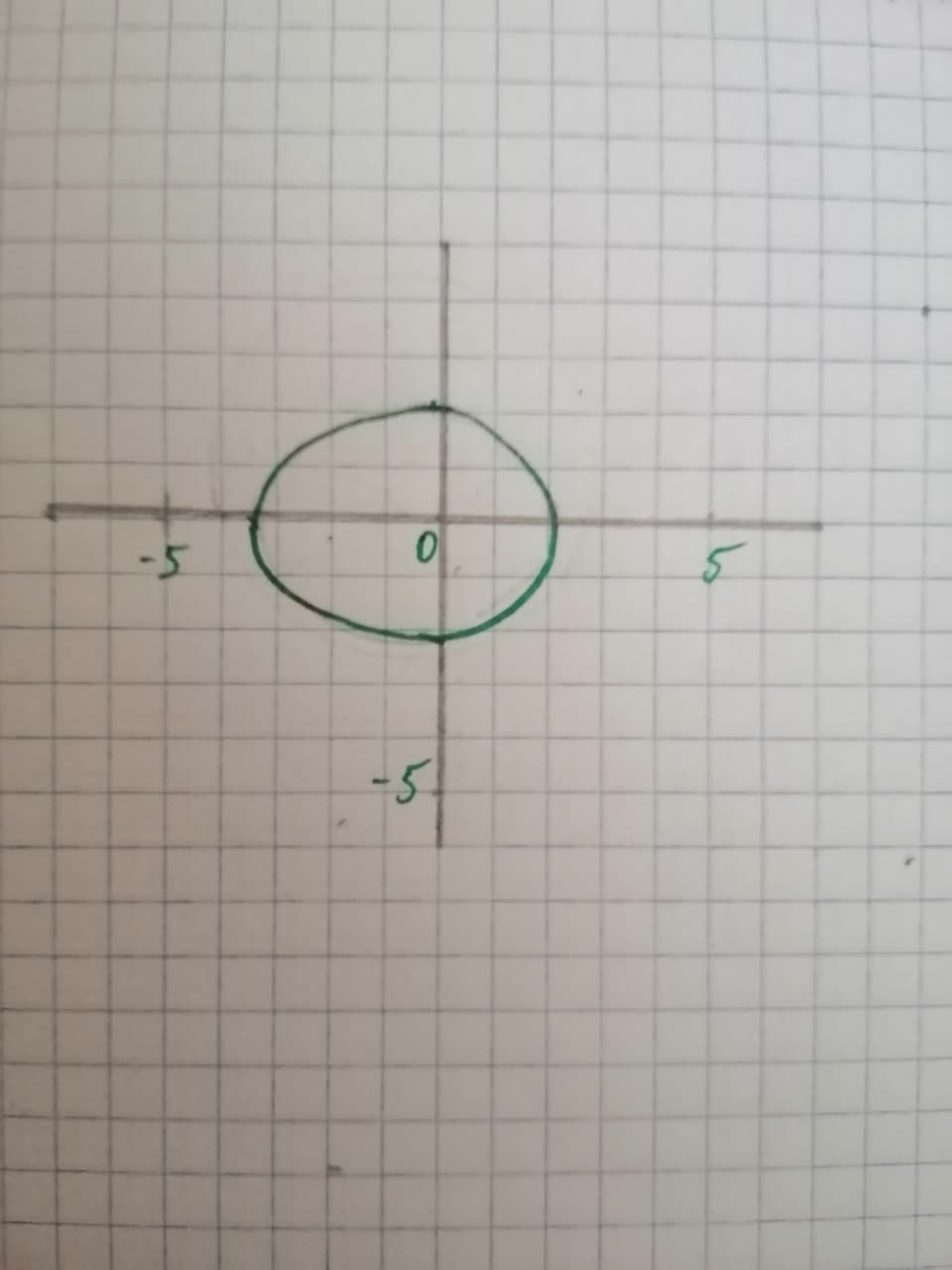#### Didn’t find what you are looking for?

Question# Polar equations for conic sections Graph the following conic sections, labeling vertices, foci, directrices, and asymptotes (if they exist). Give the eccentricity of the curve. Use a graphing utility to check your work. r= frac{10}{5 + 2 cos theta}

Conic sections
ANSWEREDPolar equations for conic sections Graph the following conic sections, labeling vertices, foci, directrices, and asymptotes (if they exist). Give the eccentricity of the curve. Use a graphing utility to check your work. $$\displaystyle{r}=\ {\frac{{{10}}}{{{5}\ +\ {2}\ {\cos{\theta}}}}}$$2021-03-08
Step 1 GIven polar equation is: $$\displaystyle{r}=\ {\frac{{{10}}}{{{5}\ +\ {2}\ {\cos{\theta}}}}}$$ The graph of the given polar equation is:Step 2 Therefore the given polar equation represents an ellipse with major axis as the x-axis, center at $$\displaystyle{\left(-{1},\ {0}\right)}.$$
$$\displaystyle{r}=\ {\frac{{{10}}}{{{\left({5}\ +\ {2}\ {\cos{\theta}}\right\rbrace}}}}$$
$$\displaystyle{r}=\ {\frac{{{2}}}{{{\left[{1}\ +\ {\left({\frac{{{2}}}{{{5}}}}\right)}\ {\cos{\theta}}\right]}}}}$$
Eccentricity $$\displaystyle{e}=\ {\frac{{{2}}}{{{5}}}}\ {<}\ {1}$$</span> Therefore the given equation represents an ellipse. Now we get, $$\displaystyle{2}={e}{p}$$
$$\displaystyle{2}=\ {\left({\frac{{{2}}}{{{5}}}}\right)}{p}$$
$$\displaystyle{p}={5}$$ The directrix is $$\displaystyle{y}={5}.$$